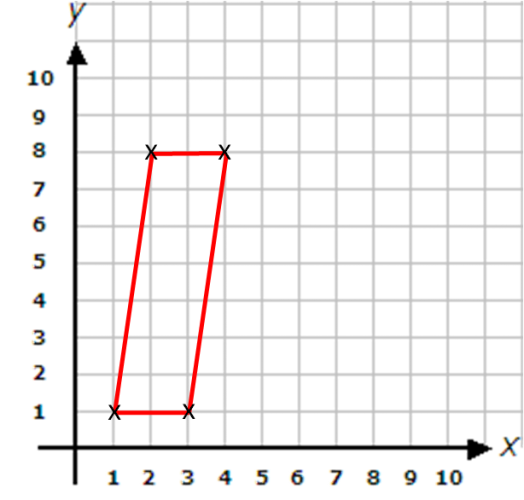For full tracking and unlimited access to thousands of activities for £1 today.

• ## Accessibility

Open the accessibility toolbar to change fonts and contrast, access a dictionary, use a ruler and more

In this worksheet, plot points on a set of x and y-coordinate axes, and connect the points to form simple shapes.

Know the following shapes:

Isosceles triangle, right-angled triangle, square, rectangle, parallelogram.

Remember that the x-coordinate is plotted before the y-coordinate.

Example

Join (1, 1) to (2, 8).

Join (2, 8) to (4, 8).

Join (4, 8 ) to (3, 1).

Join (3, 1) to (1, 1).

Which shape has been drawn?